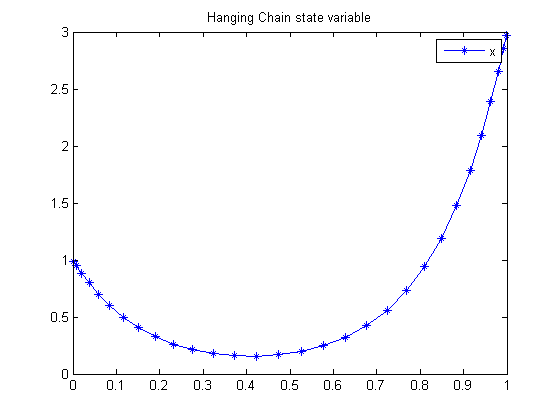49  Hanging Chain

Benchmarking Optimization Software with COPS Elizabeth D. Dolan and Jorge J. More ARGONNE NATIONAL LABORATORY

49.1  Problem Formulation

Find x(t) over t in [0; 1 ] to minimize

J =
 1 0
x*
 √
1+(
 dx dt
)2
dt

subject to:

 1 0

 √
1+(
 dx dt
)2
dt = 4
 x0 = 1
 x1 = 3

Reference: 

49.2  Problem setup

toms t
p = tomPhase('p', t, 0, 1, 30);
setPhase(p);

tomStates x

% Initial guess
a = 1; b = 3;
x0 = icollocate(x == 2*abs(b-a)*t.*(t-2*(0.25+(b<a)*0.5))+1);

% Constraints
con = {initial(x) == a
final(x) == b
integrate(sqrt(1+dot(x).^2)) == 4};

% Objective
objective = integrate(x.*sqrt(1+dot(x).^2));

49.3  Solve the problem

options = struct;
options.name = 'Hanging Chain';
solution = ezsolve(objective, con, x0, options);
t = subs(collocate(t),solution);
x = subs(collocate(x),solution);
Problem type appears to be: con
Starting numeric solver
===== * * * =================================================================== * * *
TOMLAB - Tomlab Optimization Inc. Development license  999001. Valid to 2011-02-05
=====================================================================================
Problem: ---  1: Hanging Chain                  f_k       5.068480111000088300
sum(|constr|)      0.000000000009249268
f(x_k) + sum(|constr|)      5.068480111009337800
f(x_0)      4.742150260697741300

Solver: snopt.  EXIT=0.  INFORM=1.
SNOPT 7.2-5 NLP code
Optimality conditions satisfied

FuncEv  295 GradEv  293 ConstrEv  293 ConJacEv  293 Iter  244 MinorIter  279
CPU time: 0.484375 sec. Elapsed time: 0.484000 sec.

49.4  Plot result

figure(1)
plot(t,x,'*-');
legend('x');
title('Hanging Chain state variable');